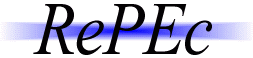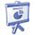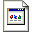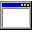Home

Testing the nullity of GARCH coefficients : correction of the standard tests and relative efficiency comparisons

Christian Francq and Jean-Michel Zakoïan
Keywords: Asymptotic efficiency of tests, Boundary, Chi-bar distribution, GARCH model, Quasi Maximum Likelihood Estimation, Local alternatives
Abstract: This article is concerned by testing the nullity of coefficients in GARCH models. The problem is non standard because the quasi-maximum likelihood estimator is subject to positivity constraints. The paper establishes the asymptotic null and local alternative distributions of Wald, score, and quasi-likelihood ratio tests. Efficiency comparisons under fixed alternatives are also considered. Two cases of special interest are: (i) tests of the null hypothesis of one coefficient equal to zero and (ii) tests of the null hypothesis of no conditional heteroscedasticity. Finally, the proposed approach is used in the analysis of a set of financial data and leads to reconsider the preeminence of GARCH(1,1) among GARCH models. The quasi-maximum likelihood estimator (QMLE), which is the most widely-used estimator for GARCH models, possesses a non standard asymptotic distribution when the true parameter has zero coefficients. It follows that tests currently implemented in softwares, such as the \$t\$-ratio test, the Wald test or the Likelihood Ratio (LR) test, are not valid for testing that some GARCH coefficients are equal to zero. For any sequence of local parameters tending to the boundary of the parameter space at the rate \$n^{1/2}\$, the asymptotic distribution of the QMLE is established. This allows to correct the asymptotic critical values of the above-mentioned tests and to compare their local asymptotic powers. We give conditions under which the modified versions of the Wald and LR tests are locally asymptotically optimal for testing the nullity of one coefficient, and we show that these tests dominate the usual two-sided score test. For testing that the ARCH coefficients are all equal to zero, we show that a one-sided version of the score test enjoys the property of being locally asymptotically most stringent somewhere most powerful. We also compute and compare the Bahadur slopes of several conditional homoscedasticity tests, showing that the asymptotic performance of a given test strongly depends on the efficiency concept (e.g. Bahadur or Pitman) chosen.RePEc working paperJournal of the American Statistical Association , 104, 313-324, 2009.
Slides of a talk given atMilan, August 27, 2008
R code used to obtain Table 4 of the MPRA preprint :program and :zip file of the series of the daily returns (obtained from yahoo).
Fortran code used in Table 6 of the MPRA preprint :F90 code for the Absoft compiler (sorry, but modifications are required with other fortran compilers) ,a file required by the program (the output corresponds to n=500 q=3 in the second part of the table),another file required by the program (but the code can be easily modified to get ride of that),executable file